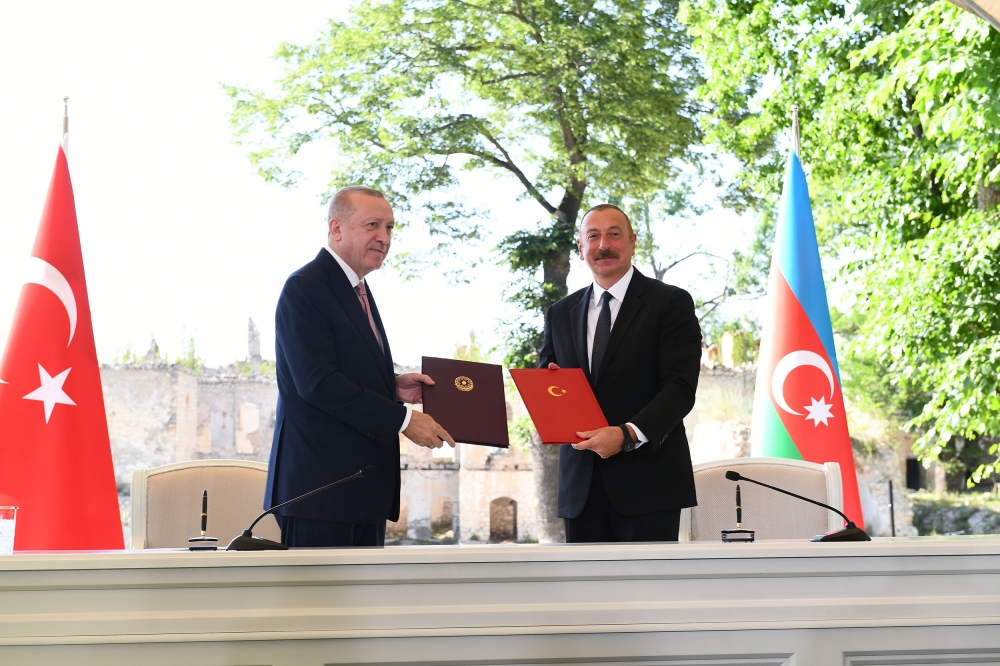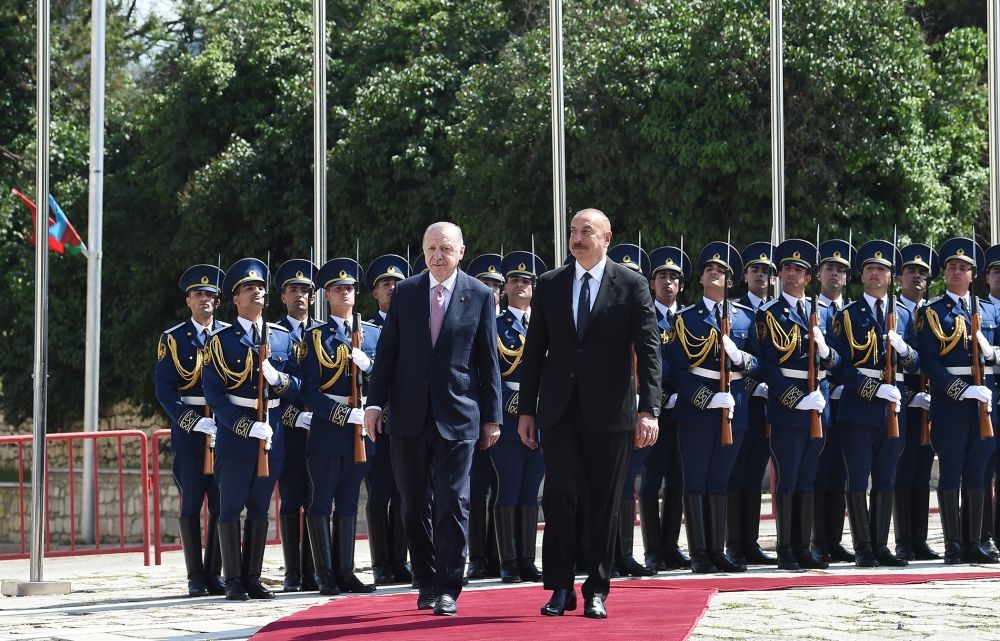Riyaziyyat və Mexanika İnstitutu
Azərbaycan Milli Elmlər Akademiyası

## Qarabağ xəbərləri### Şuşada Heydər Əliyev Fondunun təşkilatçılığı ilə “Musiqi irsi və Qarabağ atları Cıdır düzündə” adlı kompozisiya təqdim olunub### Azərbaycan ilə Türkiyə arasında müttəfiqlik münasibətləri haqqında Şuşa Bəyannaməsi imzalanıb## Department of “Equations of mathematical physics”Head of the department: Rauf Veli oglu Guseynov Doctor of physico-mathematical sciences, professor, corresponding member of NASA Tel: (+994 12)  5387250 E-mail: raufvh@yahoo.com, rauf.huseynov@imm.az Number of employees: 12 The basic scientific direction of the department: On-valued solvability of different boundary value problems for divergent and non-divergent structure linear and nonlinear quasi-elliptic equations and non-stationary equations with quasi-elliptic part; investigation of quality properties of solutions of nonlinear pseudo-hyperbolic equations; studying of direct and inverse      problems. The obtained basic scientific results: Negative spectra of quasi-elliptic                            equations were studied, their  number was estimated; the results expressing quality properties of divergent and non-divergent structure second order degenerate and non-degenerate elliptic and parabolic equations were obtained; equivalence of Wiener and Petrovsky type criteria of  regularity of the point of a boundary value problem for parabolic equations was proved; quality properties of solutions of non-divergent structure, discontinuous coefficient parabolic equations were studied; ”conditional” well-posedness of inverse problems with coefficient for linear, nonlinear, quasi-linear parabolic equations and system of equations was studied; quality properties of the solutions of a class of pseudo-hyperbolic and pseudo-parabolic equations were investigated; parabolic potentials were estimated in singular domains; asymptotics of the solutions of nonlinear equations near the singular point was studied; Poincare inequality for second order quasi-linear elliptic equations was proved; the questions of existence and uniqueness of solutions of the Dirichlet and Neumann problem for discontinuous coefficient Cordes type linear and quasi-linear elliptic equations were studied; theorems on a removable singularity of Carleson type for degenerate equations were proved; theorems on a removable singularity and theorems on the qualitative properties for p-Laplacian type quasi-linear equations with degenerating principal part were proved; Poincare-Sobolev and Hardy type uniform and non-uniform inequalities were proved; weighted Hardy inequalities in the Lebesgue spaces with a variable exponent were proved; existence of global solutions of semi-linear elliptic and parabolic type equations were studied, exact estimations for the existence of solutions were found; asymptotics of solutions satisfying the Neumann condition near the infinity was studied; uniqueness of solution of linear ordinary differential and partial equations without boundary condition was studied; behavior of Zaremba problem for second order degenerate elliptic equations in the boundary was studied, regularity of congruence point in special spherical layers was investigated.
Azərbaycanda COVID-19 ilə bağlı statistika
• Virusa yoluxan

335521

• Sağalan

329599

• Yeni yoluxan

0

• Aktiv xəstə

959

• Ölüm halı

4963

• Test edilib

3,680,977